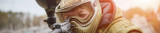## Refine Results

293 Results

V-Force

\$84.95
• Sale 18%

V-Force

Was: \$84.95
Now: \$69.95
• Sale 12%

V-Force

Was: \$124.95
Now: \$109.95
• Sale 12%

V-Force

Was: \$124.95
Now: \$109.95
• Sale 12%

V-Force

Was: \$124.95
Now: \$109.95
• Sale 14%

V-Force

Was: \$109.95
Now: \$94.95
• Sale 14%

V-Force

Was: \$109.95
Now: \$94.95
• Sale 14%

V-Force

Was: \$109.95
Now: \$94.95
• Sale 12%

V-Force

Was: \$124.95
Now: \$109.95

V-Force

\$124.95

V-Force

\$124.95

V-Force

\$124.95

V-Force

\$124.95

V-Force

\$99.95

V-Force

\$109.95

V-Force

\$109.95

V-Force

\$109.95

V-Force

\$124.90

V-Force

\$124.90

V-Force

\$124.90

V-Force

\$124.90

V-Force

\$124.90
• Sale 5%

V-Force

Was: \$99.90
Now: \$94.95

V-Force

\$124.90

V-Force

\$124.90

V-Force

\$124.90

V-Force

\$124.90

V-Force

\$84.95
• Sale 15%

V-Force

Was: \$129.99
Now: \$109.95
• Sale 46%

V-Force

Was: \$119.95
Now: \$64.95
• Sale 33%

V-Force

Was: \$119.95
Now: \$79.95
• Sale 42%

V-Force

### V-Force Grill Mask - SE White/Blue

star star star star star

Was: \$119.95
Now: \$69.95
• Sale 29%

Was: \$119.95
Now: \$84.95
• Sale 15%

V-Force

Was: \$129.99
Now: \$109.95
• Sale 50%

V-Force

Was: \$139.95
Now: \$69.95
• Sale 15%

V-Force

Was: \$129.99
Now: \$109.95
• Sale 33%

Was: \$119.95
Now: \$79.95
• Sale 33%

V-Force

Was: \$119.95
Now: \$79.95

V-Force

\$124.95

V-Force

\$139.95

V-Force

\$139.95

V-Force

\$139.95

V-Force

\$139.95

V-Force

\$139.95

V-Force

\$139.95

V-Force

\$124.95

V-Force

\$139.95

V-Force

\$139.95

V-Force

\$139.95

V-Force

\$139.95

V-Force

\$114.95

V-Force

\$124.95

V-Force

\$139.95

V-Force

\$139.95

V-Force

\$139.95

V-Force

\$139.95

V-Force

\$139.95

V-Force

\$139.95

V-Force

\$124.95